# Angles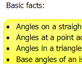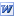Angle Reasons A sheet of the reasons required in GCSE exams as suggested in the June 2011 Edexcel examiners report. Learn them. PDF here.Polygon Formula The basic four formula required when dealing with angles in polygons. Grid for students to complete for themselves. Regular polygons for students to split into triangles.Polygons Angles List + Formula List of polygons, total interior angle, each interior (if regular), total exterior angle + rules for n sides. Grid for students to complete for themselves.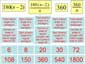Angles in Polygons A single ppt slide for pupils to copy the statements and match up the answers. The yellow boxes at the top remind pupils of the formulae they need when finding total angles in polygons and each angle of regular polygons. Interactivity could be included by getting pupils to draw lines on the IWB to match up the various statements.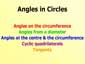Circle Theorems A talk through show of the 5 'angles in circles' facts that pupils need to know.Circle Theorems Crib sheet of those all important Angles In Circles. PDF here.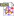Cabri File: Ciircle Theorems - Angles at CentreCabri File: Circle Theorems - Angles on Circumference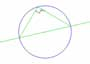Cabri File: Circle Theorems - Angles on Diameter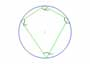Cabri File: Circle Theorems - Cyclic QuadrilateralsCabri File: Circle Theorems - Tangents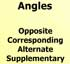Angles on Parallel Lines A talk through show of the 4 'angles on parallel lines' factsCabri File: Angles on Parallel Lines